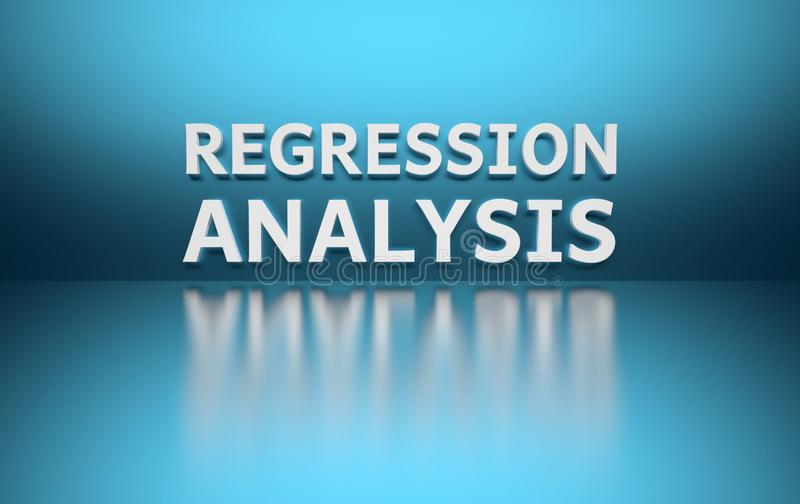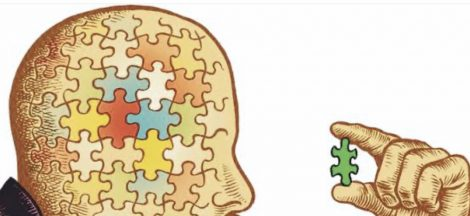Regression analysis is a predictive modelling technique that assesses the relationship between a dependent (goal/target) variable and one or more independent variables. Forecasting, time series modelling, determining the relationship between variables, and predicting continuous values can all be done using regression analysis.

Regression analysis shows the relationship between two or more variables. It is a way to find trends in data. It shows causality and can be used to forecast or predict. As an example, you might guess that there is a connection between how much you eat and how much you weigh; regression analysis can help you quantify that. If you have been putting on weight over the last few years, regression will help you predict how much you will weigh in five years’ time if you continue to add weight at the same rate.

Regression analysis convolves around simple algorithms, which are often used in finance, investing, and others. While an algorithm is a step-by-step process that goes through the data, regression analysis simply looks backward with these data to understand the pattern. When the pattern is understood, it is then easier to understand it going forward. The basis of the algorithm is regression analysis.

Types of Regression Models

We have two main types of regression analysis. They are simple regression and multiple regression.

Simple regression           –                         Linear and Non-linear

Multiple Regression       –                         Linear and Non- linear

Simple regression model: This is a model that assesses the relationship between a dependent variable and an independent variable. The simple model can be expressed using the following equation:          Y = a + bX + ϵ

Where, Y       –  Dependent or target variable

X       – Independent or explanatory or predictor variable

a        – Intercept

b        – Slope

Multiple Linear Regression: This is used to determine the relationship between three or more variables: the dependent variable and at least two independent variables. The multiple regression equation looks complex but is similar to the single variable linear regression equation. There are also two types of multiple regressions: linear and nonlinear. It can be expressed by the following equation:

Y = a + bX1 + cX+ dX3 + ϵ

Where,

Y            –             dependent variable

X1, X2, X3, –         independent variables

a            –             intercept

b,c,d,    –             slopes

ϵ            –             Residual (error)

Regression analysis does the following three things. It shows relationships, shows causality, and can be used to forecast or predict. In regression, you must be clear on what is your dependent variable and independent variable. For regression to make sense, certain assumptions must be considered and included.

In analysis, before you interpret numbers you must have a good understanding of your domain. It is important for managers to understand their domain first before applying these tools and techniques to areas under their control. It has a positive and negative relationship but can also have a cost and effect impact.

There are situations where although you keep increasing labour, production keeps reducing, why? Maybe labour is not the issue at hand. It could be a diminishing return issue. Independent variable does not depend on anything. It can find solutions on its own but dependent variables cannot be determined with the independent.## Lessons learned from the movie “Eye in the Sky”

in## Learning the act of thinking

in